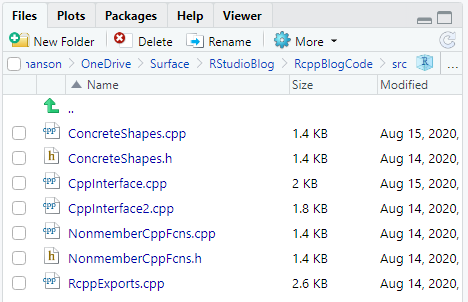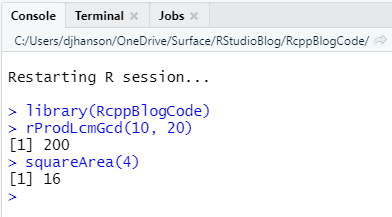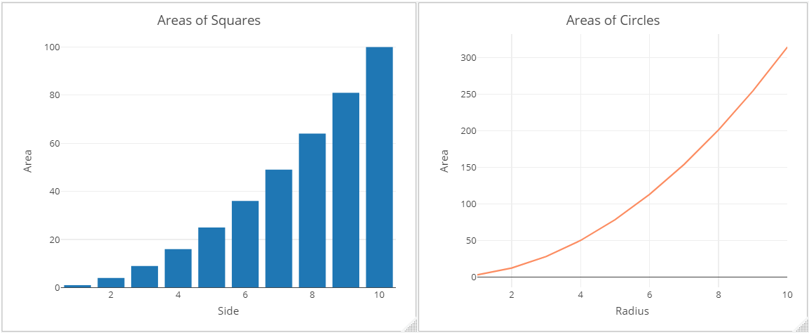# R Package Integration with Modern Reusable C++ Code Using Rcpp - Part 5

by Daniel Hanson

Daniel Hanson is a full-time lecturer in the Computational Finance & Risk Management program within the Department of Applied Mathematics at the University of Washington.

In the previous post, we went through the build processes for a simple package containing a single C++ .cpp file, using the rcpp_hello_world.cpp example that is included by default when one creates a new R package using Rcpp in RStudio.

What we’ll do now is examine a more realistic case which involves multiple reusable C++ files as well as the C++ interface files that export functions to R. For this example, you should download the C++ files from this GitHub repository. In addition, you should download a set of test functions in R, here (RStudioBlogTests.R). Please note that this file is not part of the package code; we will discuss this shortly.

## Building an Rcpp Package with Reusable C++

The code you have downloaded from the first link is the same as that referenced in Part 3 of this series. You will now create an Rcpp package project in RStudio and import this code.

### Create an RStudio Project

This procedure is the same as what we covered in the previous post. For convenience later, I suggest you name your project RcppBlogCode. Make sure to modify the NAMESPACE file as we discussed before. Also, while not mandatory, you may find it simpler to delete the rcpp_hello_world.cpp file; we will not use it in this example.

### Import the C++ Code into the Project

Next, copy or move the code you downloaded from the /src subdirectory on GitHub into the /src subdirectory of your RStudio project. When this is done, you should see the files present in this location in the files pane in RStudio:Note that this source code contains:

• Declaration (header files) for the reusable C++ code

• NonmemberCppFcns.h: Declarations of nonmember functions
• ConcreteShapes.h: Declarations of the Square and Circle classes
• Files containing implementations of functions and classes in the reusable C++ code

• NonmemberCppFcns.cpp: Nonmember function implementations
• ConcreteShapes.cpp: Square and Circle class implementations
• C++ files that call reusable code and export functions to R (.cpp files only); each exported function is indicated by the // [[Rcpp::export]] tag

• CppInterface.cpp: Calls nonmember functions in the code base
• CppInterface2.cpp: Creates and uses instances of classes in the code base

### Build and Run Functions in the R Package

Next, let’s build this package, just as we did for our simpler example in the previous post. Select Clean and Rebuild from the Build menu at the top of the RStudio IDE. When complete, R will restart, and the RcppBlogCode package will be loaded into your R session. You can now call functions from this package in the console; e.g. ,

• Calculate the product of the LCM and GCD of 10 and 20: rProdLcmGcd(10, 20)
• Calculate the area of a square with length 4: squareArea(4)Try package functions in console

Congratulations again! You have now successfully imported resuable C++ code, independent of R and Rcpp, and used it via an interface as an R function in your generated package. However, this of course is not a realistic scenario. What we really want is to use this package in a regular and independent R session.

## Use the Package Independently of the Rcpp Project

So far, you have built an R package containing C++ code, and you have called a couple of functions from the console, but that of course is not what a real R user does. What you’ll want to do is open a new (and empty) RStudio instance. As the RcppBlogCode package is installed on your machine, all you need to do is load it as you would any other R package:

library(RcppBlogCode)

Next, open up the RStudioBlogTests.R test file that you downloaded at the outset. Again, make sure this file is located outside of the Rcpp package directory structure. You can now use the package functions that call the C++ nonmember functions internally in the package:

rAdd(531, 9226)

(x <- c(5:1))
rSortVec(x)

rProdLcmGcd(10, 20)


You can also run the R functions that create instances of the Square and Circle classes in the C++ code:

squareArea(4)
circleArea(1)

The actual mathematics here are obviously nothing exciting, but you should now see that you have a blueprint that takes in independent and reusable C++ source code, wraps its functionality in C++ interface functions that get exported by Rcpp, and allows you to use these exported functions just as you would any other R package functions.

Perhaps more interesting is to see how you can take the results of these package functions and use them in other R functions and packages. For this next example, you will need to install and load the plotly package. We will define vectors of square sides and circle radii, and compute the corresponding areas using our package functions. This is again nothing earth-shattering, but we can then use them in plotly to generate some reasonably professional quality graphs:

library(plotly)

squareSides <- c(1:10)

squareAreas <- sapply(squareSides, squareArea)

### Use plotly to visualize some results:
dfSq <- matrix(data=c(squareSides, squareAreas), nrow=length(squareSides), ncol=2)
dfSq <- as.data.frame(dfSq)
colnames(dfSq) = c("Side", "Area")
rownames(dfSq) = NULL
plot_ly(dfSq, x = ~Side, y = ~ Area, type = "bar") %>%
layout(title="Areas of Squares")

dfCirc <- matrix(data=c(circleRadii, circleAreas), nrow=length(squareSides), ncol=2)
dfCirc <- as.data.frame(dfCirc)
rownames(dfCirc) = NULL
plot_ly(dfCirc, x = ~Radius, y = ~ Area, type = 'scatter',
mode = 'lines', color = 'red') %>%
layout(title="Areas of Circles")Using results from package in Plotly graphs

Remark 1: If you wish to go back and modify the package code, you will need to close your R session that is using the package; otherwise, the code will not build.

Remark 2: As discussed last time, you can also export the package to a binary and deploy it on another machine. In general, this allows you to design and build your own Rcpp R package, and deploy it for your colleagues to use, if you wish.

## Summary

You have now seen how to import standard and reusable C++ code into an R package using Rcpp, use the package in R, and deploy it as a binary. The one remaining topic to cover is documentation, which we will take up next time.

Share Comments · · · · · ·